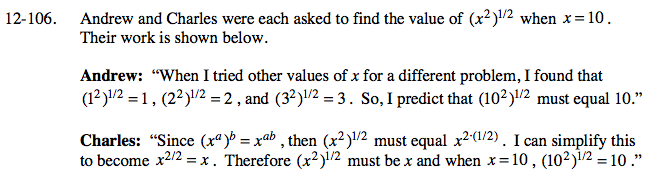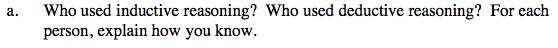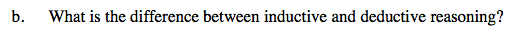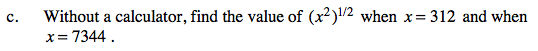### Home > CAAC > Chapter 12 > Lesson 12.4.4 > Problem12-106

12-106.
1. Andrew and Charles were each asked to find the value ofwhen x = 10. Their work is shown below. Homework Help ✎

Andrew: “When I tried other values of x for a different problem, I found that,, and. So, I predict thatmust equal 10.”

Charles: “Since (xa)b = xab, thenmust equal. I can simplify this to become. Thereforemust be x and when x = 10,.”

1. Who used inductive reasoning? Who used deductive reasoning? For each person, explain how you know.

2. What is the difference between inductive and deductive reasoning?

3. Without a calculator, find the value ofwhen x = 312 and when x = 7344.Andrew used inductive reasoning because he extended a pattern; Charles used deductive reasoning because he based his reasoning on properties of exponents.What are the definitions of inductive and deductive?Follow the reasoning of Andrew and Charles.